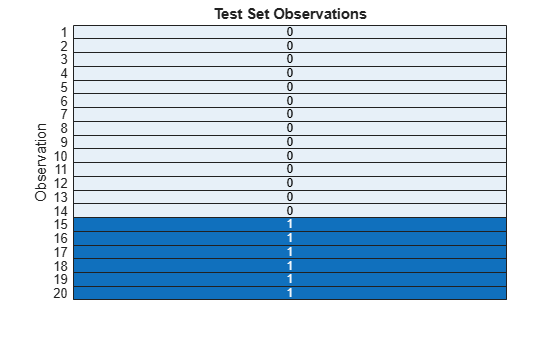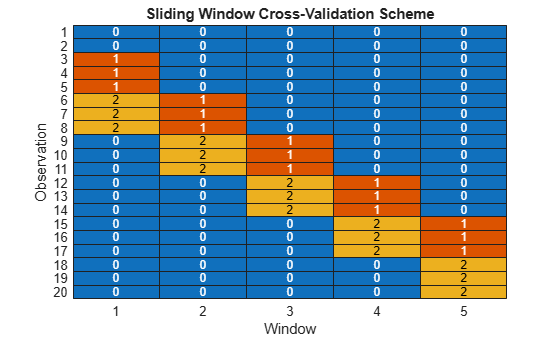# test

Test indices for time series cross-validation

## Syntax

``idx = test(c)``
``idx = test(c,i)``

## Description

example

````idx = test(c)` returns the test indices `idx` for a `tspartition` object `c` of type `'holdout'`. That is, the logical vector `idx` specifies the observations in the test set.```

example

````idx = test(c,i)` returns the test indices for window `i` of a `tspartition` object `c` of type `'expanding-window'` or `'sliding-window'`. That is, the logical vector `idx` specifies the observations in test set `i`.If `c.Type` is `'expanding-window'`, then the training set size expands with each window while the test set size remains fixed.If `c.Type` is `'sliding-window'`, then both the training set size and the test set size are fixed.```

## Examples

collapse all

Identify the observations in the test set of a `tspartition` object for holdout validation.

Use 30% of 20 time-dependent observations to create a test set. The corresponding training set contains the remaining observations.

`c = tspartition(20,"Holdout",0.30);`

Find the test set indices. A value of 1 (`true`) indicates that the corresponding observation is in the test set. A value of 0 (`false`) indicates that the corresponding observation is in the training set.

`testIndices = test(c);`

Visualize the observations in the test set by using a heat map.

```h = heatmap(double(testIndices),ColorbarVisible="off"); h.XDisplayLabels = ""; ylabel("Observation") title("Test Set Observations")```The observations in light blue (with a value of 0) are in the training set, and the observations in dark blue (with a value of 1) are in the test set. When you use holdout validation for time series data, the latest observations (in this case, observations 15 through 20) are in the test set.

Identify the observations in the training sets and test sets of a `tspartition` object for sliding window cross-validation.

Use 20 time-dependent observations to create five training sets and five test sets.

`c = tspartition(20,"SlidingWindow",5);`

Find the training set indices for the five windows. A value of 1 (`true`) indicates that the corresponding observation is in the training set for that window.

```trainWindows = zeros(c.NumObservations,c.NumTestSets); for i = 1:c.NumTestSets trainWindows(:,i) = training(c,i); end```

Find the test set indices for the five windows. A value of 1 (`true`) indicates that the corresponding observation is in the test set for that window.

```testWindows = zeros(c.NumObservations,c.NumTestSets); for i = 1:c.NumTestSets testWindows(:,i) = test(c,i); end```

Combine the training and test set indices into one matrix where a value of 1 indicates a training observation and a value of 2 indicates a test observation.

`data = trainWindows + 2*testWindows;`

Visualize the different sets by using a heat map.

```colormap = lines(3); heatmap(double(data),ColorbarVisible="off", ... Colormap=colormap); xlabel("Window") ylabel("Observation") title("Sliding Window Cross-Validation Scheme")```For each window, the observations in red (with a value of 1) are in the training set, the observations in yellow (with a value of 2) are in the test set, and the observations in blue (with a value of 0) are ignored. For example, observations 9 through 11 are test observations in window two and training observations in window three. Because of the default values for the training set size, test set size, step size, and direction for creating sliding windows, `tspartition` does not use some of the oldest observations (1 and 2) in any window.

## Input Arguments

collapse all

Time series validation partition, specified as a `tspartition` object. The validation partition type (`Type`) is `'expanding-window'`, `'holdout'`, or `'sliding-window'`.

Test set or window index, specified as a positive integer scalar. When you specify `i`, the `test` function finds the observations in test set `i`.

Data Types: `single` | `double`

## Output Arguments

collapse all

Indices for test set observations, returned as a logical vector. A value of 1 (`true`) indicates that the corresponding observation is in the test set. A value of 0 (`false`) indicates that the corresponding observation is in a different set, such as the training set.

## Version History

Introduced in R2022b